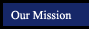mathematical logic symmetry and similarity points, lines and planes angles parallel lines triangles quadrilaterals polygons circles 3-Dimensional Geometry conclusion angle of rotation between acute angle alternate interior angles acute triangle consecutive angles decagon center base conditional statement contraction colinear angle alternate exterior angles altitude of a triangle consecutive sides exterior angle circle cylinder congruent dilation congruent segments angle bisector corresponding angles base of a triangle opposite angles hexagon circumscribed angle equation of a plane conjecture image coplanar angle measure consecutive interior angles height opposite sides interior angle concentric circles lateral surface area contrapositive line of symmetry intersect complementary linear pair hypotenuse kite nonagon diameter parallel to a plane converse midpoint congruent angles same side interior angles legs parallelogram octagon inscribed angle prism counterexample reflection line linear pair same side exterior angles median of a triangle quadrilateral pentagon radius pyramid definition rotation parallel obtuse angle transversal incenter of a triangle rectangle regular polygon tangent sphere deductive reasoning similar polygons perpendicular right angle vertical angles obtuse triangle rhombus surface area hypothesis transformation perpendicular bisector side of an angle right triangle square volume inductive reasoning translation plane supplementary sides trapezoid xy plane inverse point vertex of an angle vertex (verticies) xz plane postulate segment bisector vertical angles yz plane Reflexive Property skew Symmetric Property Transitive Property theorem undefined term